## Selina Publishers Concise Mathematics Class 7 ICSE Solutions Chapter 5 Exponents (Including Laws of Exponents)

Selina Publishers Concise Mathematics Class 7 ICSE Solutions Chapter 5 Exponents (Including Laws of Exponents)

### Exponents Exercise 5A – Selina Concise Mathematics Class 7 ICSE Solutions

Question 1.
Find the value of:
(i) 6²
(ii) 73
(iii) 44
(iv) 55
(v) 83
(vi) 75
Solution: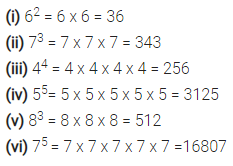Question 2.
Evaluate:
(i) 23 x 42
(ii) 23 x 52
(iii) 33 x 52
(iv) 22 x 33
(v) 32 x 52
(vi) 53 x 24
(vii) 3x 42
(ix) (5 x 4)2
Solution: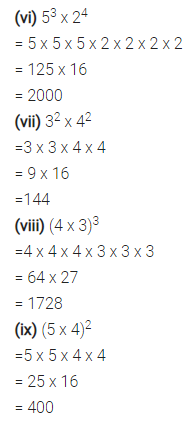Question 3.
Evaluate: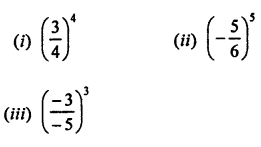Solution: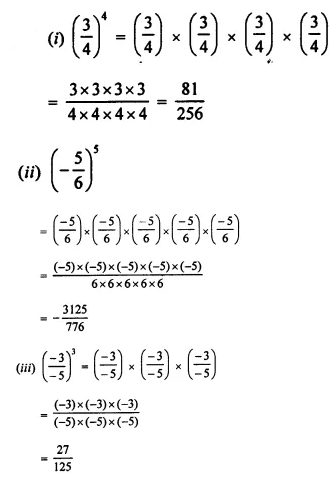Question 4.
Evaluate :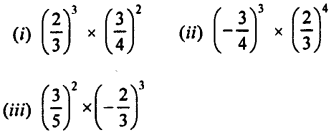Solution:Question 5.
Which is greater :
(i) 23 or 32
(ii) 25 or 52
(iii) 43 or 34
(iv) 54 or 45
Solution: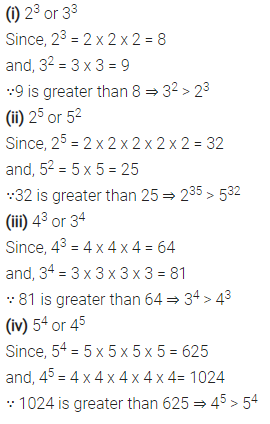Question 6.
Express each of the following in exponential form :
(i) 512
(ii) 1250
(iii) 1458
(iv) 3600
(v) 1350
(vi) 1176
Solution: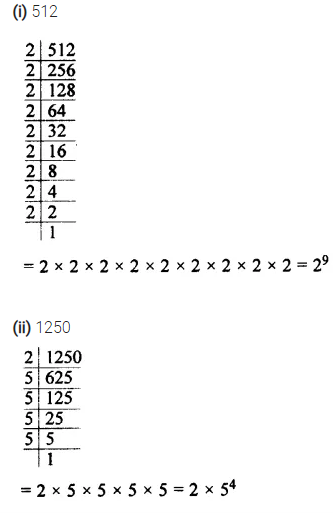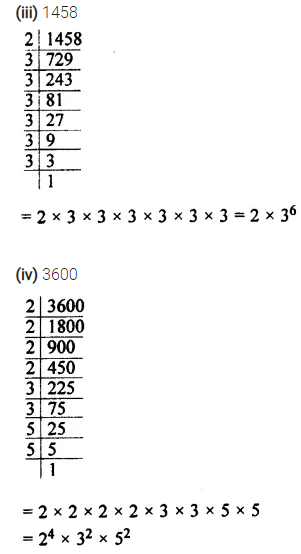Question 7.
If a = 2 and b = 3, find the value of:
(i) (a + b)2
(ii) (b – a)3
(iii) (a x b)a
(iv) (a x b)b
Solution:Question 8.
Express:
(i) 1024 as a power of 2.
(ii) 343 as a power of 7.
(iii) 729 as a power of 3.
Solution: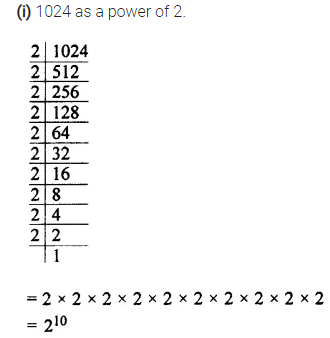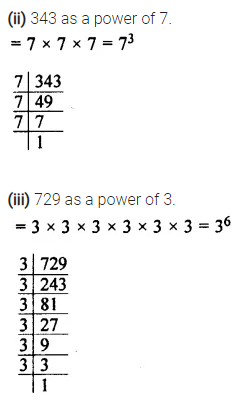Question 9.
If 27 x 32 = 3x x 2y; find the values of x and y.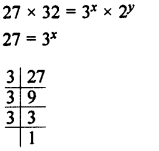Solution: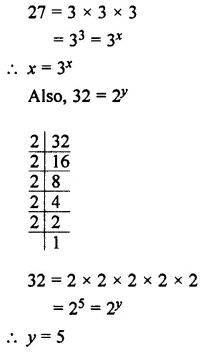Question 10.
If 64 x 625 = 2a x 5b; find :
(i) the values of a and b.
(ii) 2b x 5a
Solution: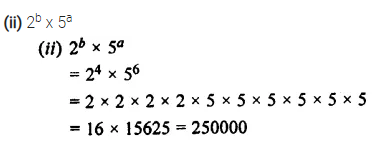### Exponents Exercise 5B – Selina Concise Mathematics Class 7 ICSE Solutions

Question 1.
Fill in the blanks:
In 52 = 25, base = ……… and index = ……….
If index = 3x and base = 2y, the number = ………
Solution:Question 2.
Evaluate:
(i) 28 ÷ 23
(ii) 2 28
(iii) (26)0
(iv) (3o)6
(v) 83 x 8-5 x 84
(vi) 5 x 53 + 55
(vii) 54 ÷ 53 x 55
(viii) 44 ÷ 43 x 40
(ix) (35 x 47 x 58)0
Solution: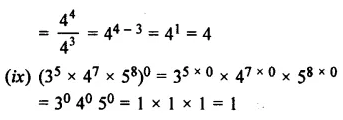Question 3.
Simplify, giving Solutions with positive index: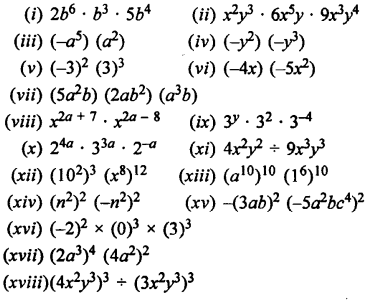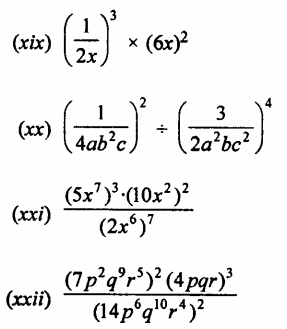Solution: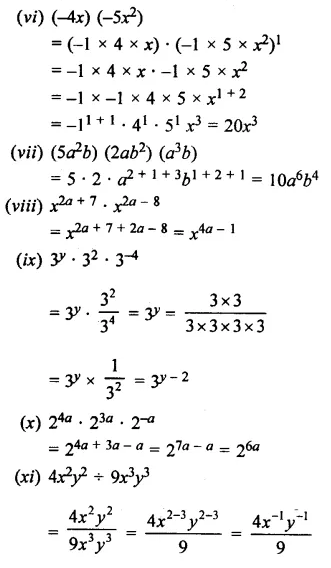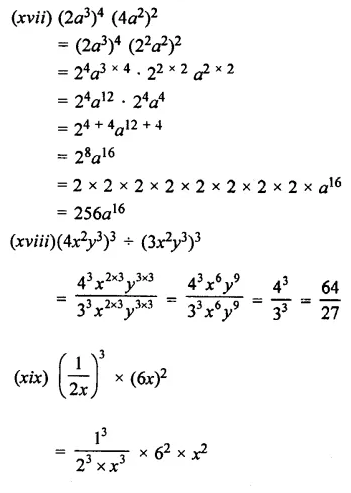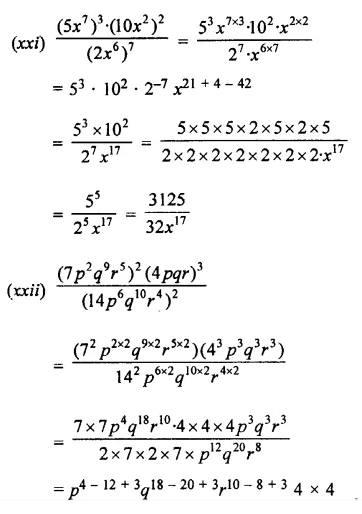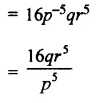Question 4.
Simplify and express the Solution in the positive exponent form :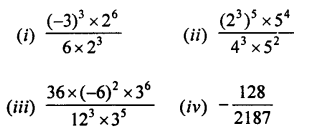Solution: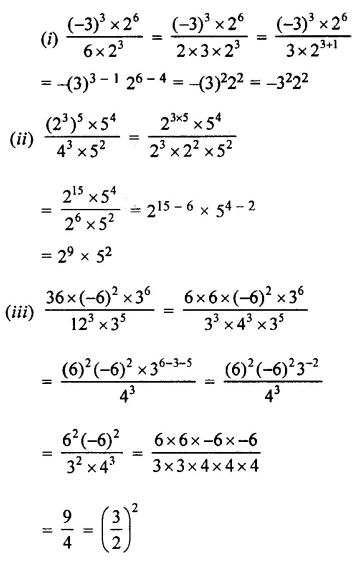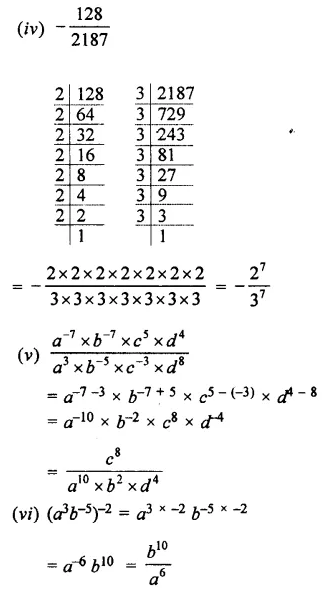Question 5.
Evaluate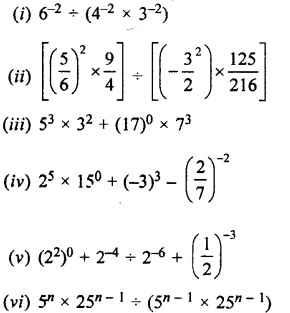Solution: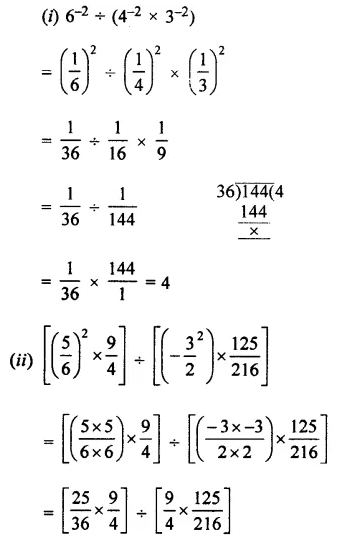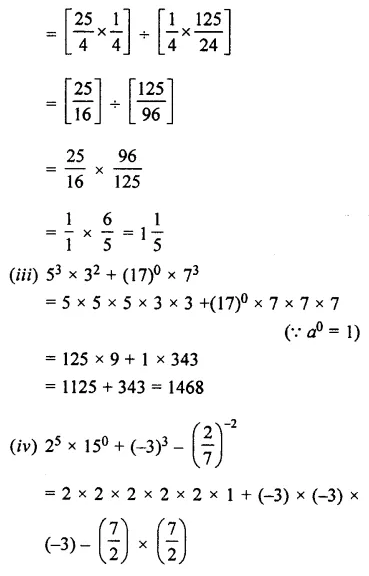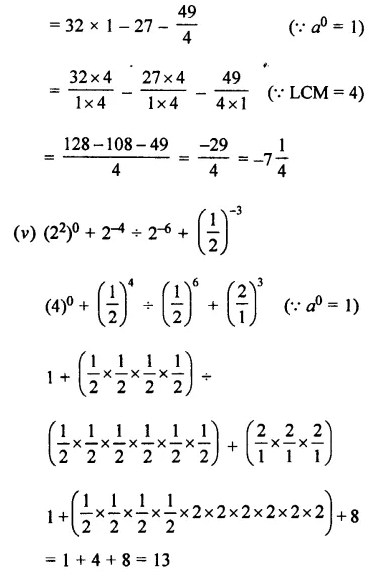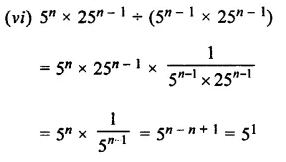Question 6.
If m2 = -2 and n = 2; find the values of:
(i) m + r2 – 2mn
(ii) mn + nm
(iii) 6m-3 + 4n2
(iv) 2n3 – 3m
Solution: